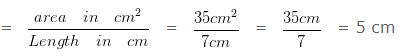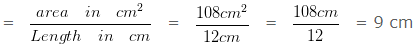# To Find Perimeter of Rectangle when Area & Length given

Question 1

The area of a rectangle is 35 cm² and its length is 7 cm. Find the perimeter of the rectangle?

Explanation

Given

Area = 35 cm²

length = 7 cmPerimeter of the rectangle = 2 x ( l + b ) cm

= 2 x ( 7 + 5 ) cm

= ( 2 x 12 ) cm

= 24 cm

Hence, perimeter of the rectangle is 24 cm.

Question 2

The area of a rectangle is 108 cm² and its length is 12 cm . Find the perimeter of the rectangle?

Explanation

Given

Area = 108 cm²

length = 12 cm